Contents >> Engineering Mathematics >> Hydro Mechanical Drives and Transmissions >> Dynamic Analysis >> Structure description of arbitrary hydro mechanical circuits >> Reduction of inertia moments and transfer attitudes

 Dynamic analysis of mechanical transmissions - Reducing of inertia moments and transfer ratios Reduction of moments of inertia and transfer ratios Let's consider the m -th site containing the following nodes: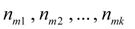, where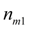– an initial node,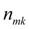– a final node. Let's enter the following designations: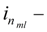a transfer ratio in node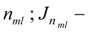the reduced moment of inertia in node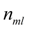. First transfer ratios in nodes of the site, reduced to initial node(thus it is obvious, that always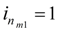) are defined: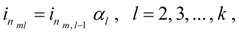(6) where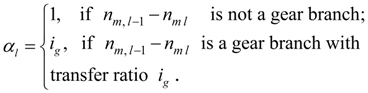(7) If the site contains branches, each of which has the final node, then transfer ratios are reduced from the formula (6) consistently on each branch. After definition of transfer ratios in all nodes of the site, moments of inertia of its elements are reduced to initial node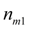: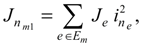(8) where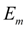– a set of elements putting together the m -th site; е – some element of the site;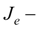moment of inertia of an element or of its part (for frictional clutch, hydro dynamic clutch or the hydraulic torque converter  – the moment of inertia according to a leading or driven part as they belong to different sites; for a gear – the moment of inertia of a branch; a shaft is considered as no inertial element);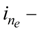the transfer ratio in node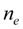of an element е . Thus, for forming of system of equations of a kind (2) all constants entering into the equations are defined, and also binding of variables and constants of these equations to external nodes of sites is realized. For definition of moments belong to the right parts of the equations (2), it is necessary to give their mathematical description in view of characteristics of drive base elements.

 < Previous Contents Next >